# impulseest

Nonparametric impulse response estimation

## Syntax

``sys = impulseest(data)``
``sys = impulseest(data,n)``
``sys = impulseest(data,n,nk)``
``sys = impulseest(___,opt)``

## Description

example

````sys = impulseest(data)` estimates an impulse response model `sys`, also known as a finite impulse response (FIR) model, using time-domain or frequency-domain data `data`. The function uses persistence-of-excitation analysis on the input data to select the model order (number of nonzero impulse response coefficients.)Use nonparametric impulse response estimation to analyze input/output data for feedback effects, delays and significant time constants.```

example

````sys = impulseest(data,n)` estimates an `n`th order impulse response model that corresponding to the time range `0:Ts:(n–1)*Ts`, where `Ts` is the data sample time.```

example

````sys = impulseest(data,n,nk)` specifies a transport delay of `nk` samples in the estimated impulse response.```

example

````sys = impulseest(___,opt)` specifies estimation options using the options set `opt`.```

## Examples

collapse all

Estimate a nonparametric impulse response model using data from a hair dryer. The input is the voltage applied to the heater and the output is the heater temperature. Use the first 500 samples for estimation.

Load the data and use the first 500 samples to estimate the model.

```load dry2 ze = dry2(1:500); sys = impulseest(ze);```

`ze` is an `iddata` object that contains time-domain data. `sys`, the identified nonparametric impulse response model, is an `idtf` model.

Analyze the impulse response of the identified model from time 0 to 1.

`h = impulseplot(sys,1);`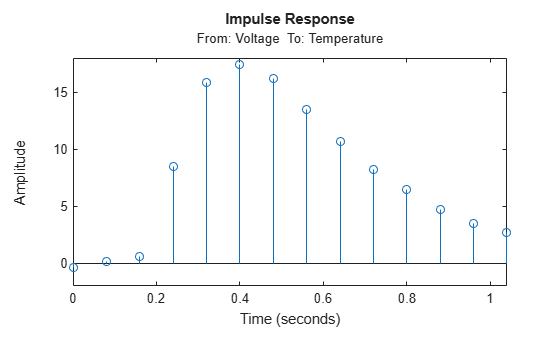Right-click the plot and select Characteristics > Confidence Region to view the region of statistically zero response. Alternatively, you can use the `showConfidence` command.

`showConfidence(h);`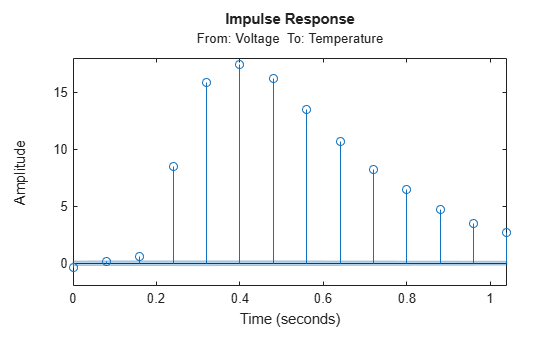The first significantly nonzero response value occurs at 0.24 seconds, or the third lag. This implies that the transport delay is 3 samples. To generate a model that imposes the 3-lag delay, set the transport delay, which is the third argument, to 3. You must also set the second argument, order `n`, to its default value of `[]` as a placeholder.

```sys1 = impulseest(ze,[],3); h1 = impulseplot(sys1,1); showConfidence(h1);```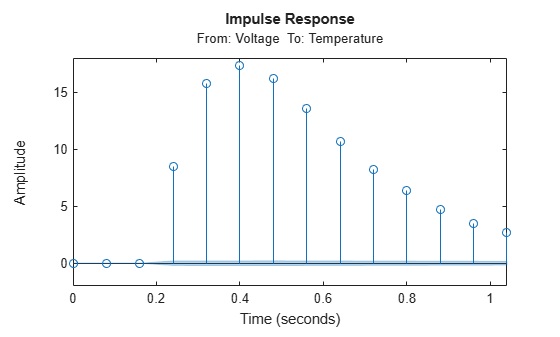The response is identically zero until 0.24s.

`load iddata3 z3;`

Estimate a 35th order FIR model.

```n = 35; sys = impulseest(z3,n);```

You can confirm the model order of `sys` by displaying the number of terms.

`nsys = size(sys.num)`
```nsys = 1×2 1 35 ```

Set `n` to `[]` so that the function automatically determines `n`. Display the model order.

```n = []; sys1 = impulseest(z3,n); nsys1 = size(sys1.Numerator)```
```nsys1 = 1×2 1 70 ```

The model order is 70. The default value for the order is `[]`, so setting the order to `[]` is equivalent to omitting the specification.

Estimate an impulse response model with transport delay of 3 samples.

If you know about the presence of delay in the input/output data in advance, use the delay value as a transport delay for impulse response estimation.

Generate data that contains a 3-sample input-to-output lag.

Create a random input signal. Construct an `idpoly` model that includes three sample delays that you implement by using three leading zeros in the B polynomial.

```u = rand(100,1); A = [1 .1 .4]; B = [0 0 0 4 -2]; C = [1 1 .1]; sys = idpoly(A,B,C);```

Simulate the model response `y` to the noise signal, using the `AddNoise` option and a sample time of 1s. Encapsulate `y` in an `iddata` object.

```opt = simOptions('AddNoise',true); y = sim(sys,u,opt); data = iddata(y,u,1);```

Estimate and plot a 20th order model with no transport delay.

```n = 20; model1 = impulseest(data,n); impulseplot(model1);```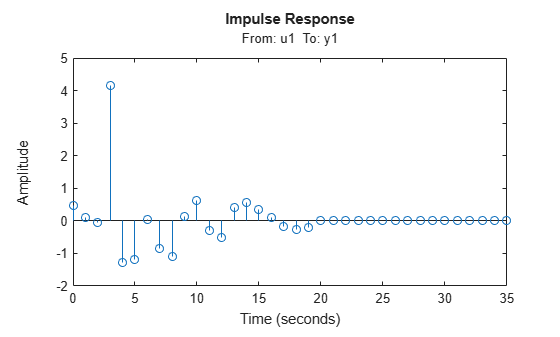The plot shows that the impulse response includes nonzero samples during the 3-second delay period.

Estimate a model with a 3-sample transport delay.

```nk = 3; model2 = impulseest(data,n,nk); impulseplot(model2)```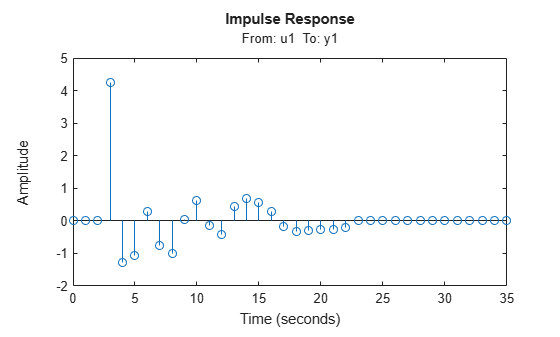The first three samples are identically 0.

Obtain regularized estimates of impulse response model using the regularizing kernel estimation option.

Estimate a model using regularization. `impulseest` performs regularized estimates by default, using the tuned and correlated kernel (`'TC'`).

```load iddata3 z3; sys1 = impulseest(z3);```

Estimate a model with no regularization.

```opt = impulseestOptions('RegularizationKernel','none'); sys2 = impulseest(z3,opt);```

Compare the impulse response of both models.

```h = impulseplot(sys2,sys1,70); legend('sys2','sys1')```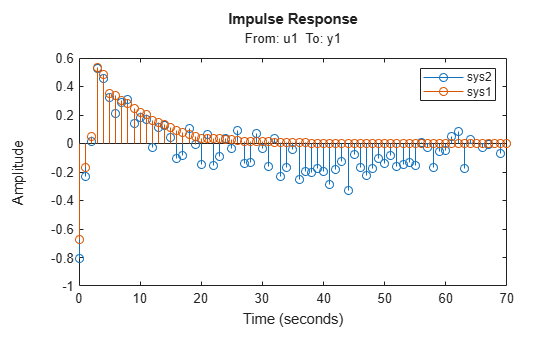As the plot shows, using regularization makes the response smoother.

Plot the confidence interval.

`showConfidence(h);`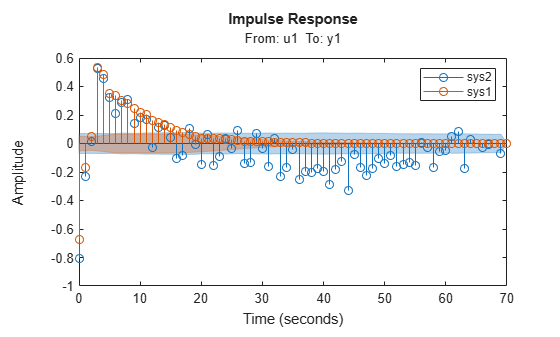The uncertainty in the computed response is reduced at larger lags for the model using regularization. Regularization decreases variance at the price of some bias. The tuning of the `'TC'` regularization is such that the variance error dominates the overall error.

`load regularizationExampleData eData;`

Recreate the transfer function model that was used for generating the estimation data (true system).

```num = [0.02008 0.04017 0.02008]; den = [1 -1.561 0.6414]; Ts = 1; trueSys = idtf(num,den,Ts);```

Obtain a regularized impulse response (FIR) model with an order of 70.

```opt = impulseestOptions('RegularizationKernel','DC'); m0 = impulseest(eData,70,opt);```

Convert the model into a state-space model and reduce the model order.

```m1 = idss(m0); m1 = balred(m1,15);```

Estimate a second state-space model directly from `eData` by using regularized reduction of an ARX model.

`m2 = ssregest(eData,15);`

Compare the impulse responses of the true system and the estimated models.

```impulse(trueSys,m1,m2,50); legend('trueSys','m1','m2');```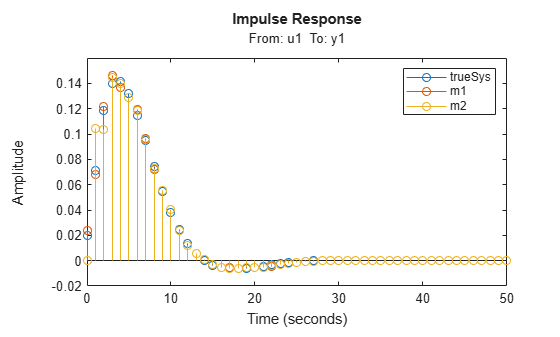The three model responses are similar.

Use the empirical impulse response to measured data to determine whether the data includes feedback effects. Feedback effects may be present when the impulse response includes statistically significant response values for negative time values.

Compute the noncausal impulse response using a fourth-order prewhitening filter and no regularization, automatic order selection, and negative lag.

```load iddata3 z3; opt = impulseestOptions('pw',4,'RegularizationKernel','none'); sys = impulseest(z3,[],'negative',opt);```

`sys` is a noncausal model containing response values for negative time.

Analyze the impulse response of the identified model.

`h = impulseplot(sys);`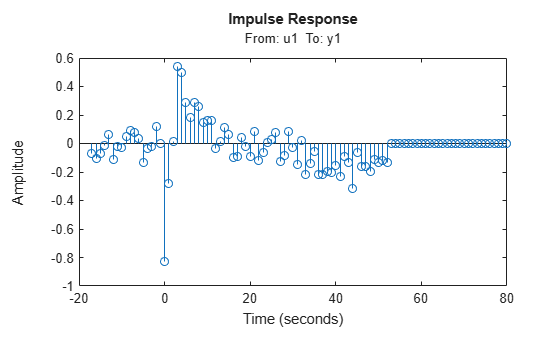View the statistically zero-response region by right-clicking on the plot and selecting Characteristics > Confidence Region. Alternatively, you can use the `showConfidence` command.

`showConfidence(h);`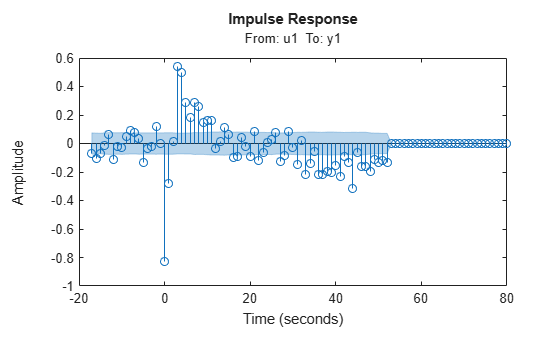The large response value at `t=0` (zero lag) suggests that the data comes from a process containing feedthrough. That is, the input affects the output instantaneously. The large response value could also indicate direct feedback, such as proportional control without some delay so that y`(t)` partly determines u`(t)`.

Other indications of feedback in the data are the significant response values such as those at -7s and -9s.

Compute an impulse response model for frequency response data.

Load the frequency response data, which contains measured amplitude `AMP` and phase `PHA` for the frequency vector W`.`

`load demofr;`

Create the complex frequency response `zfr` and encapsulate it in an `idfrd` object that has a sample time of 0.1s. Plot the data.

```zfr = AMP.*exp(1i*PHA*pi/180); Ts = 0.1; data = idfrd(zfr,W,Ts);```

Estimate an impulse response model from `data` and plot the response.

```sys = impulseest(data); impulseplot(sys)```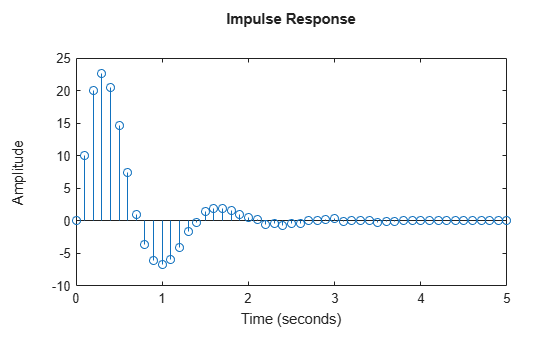Identify parametric and nonparametric models for a data set, and compare their step response.

Estimate the impulse response model `sys1` (nonparametric) and state-space model `sys2` (parametric) using the estimation data set `z1`.

```load iddata1 z1; sys1 = impulseest(z1); sys2 = ssest(z1,4);```

`sys1` is a discrete-time identified transfer function model. `sys2` is a continuous-time identified state-space model.

Compare the step responses for `sys1` and `sys2`.

```step(sys1,'b',sys2,'r'); legend('impulse response model','state-space model');```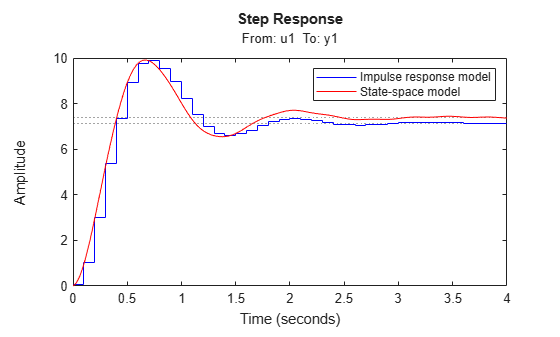## Input Arguments

collapse all

Estimation data, specified as an `iddata` object, an `idfrd`, or an `frd` (Control System Toolbox) object, with at least one input signal and a nonzero sample time.

For time-domain estimation, specify `data` as an `iddata` object containing the input and output signal values.

For frequency-domain estimation, specify `data` as one of the following:

• Frequency response data (`idfrd` object or `frd` object)

• `iddata` object with properties specified as follows:

• `InputData` — Fourier transform of the input signal

• `OutputData` — Fourier transform of the output signal

• `Domain` — ‘Frequency’

Order of the FIR model, specified as a positive integer, `[]`, or a matrix.

• If `data` contains a single input channel and output channel, or if you want to apply the same model order to all input/output pairs, specify `n` as a positive integer.

• If `data` contains Nu input channels and Ny output channels, and you want to specify individual model orders for the input/output pairs, specify `n` as an Ny-by-Nu matrix of positive integers, such that N(i,j) represents the length of impulse response from input j to output i.

• If you want the function to determine the order automatically, specify `n` as `[]`. The software uses persistence-of-excitation analysis on the input data to select the order.

Example: `sys = impulseest(data,70)` estimates an impulse response model of order 70.

Transport delay in the estimated impulse response, specified as a scalar integer, `'negative'`, or an Ny-by-Nu matrix, where Ny is the number of outputs and Nu is the number of inputs. The impulse response (input `j` to output `i`) coefficients correspond to the time span `nk(i,j)*Ts : Ts : (n(ij)+nk(i,j)-1)*Ts`.

• If you know the value of the transport delay, specify `nk` as a scalar integer or a matrix of scalar integers.

• If you do not know the delay value, specify `nk` as `0`. Once you have estimated the impulse response, you can determine the true delay from the insignificant impulse response values in the beginning portion of the response. For an example of finding true delay, see Identify Nonparametric Impulse Response Model from Data.

• To generate the impulse response coefficients for negative time values, which is useful for feedback analysis, use a negative integer. If you specify a negative value, the value must be the same across all output channels. You can also specify `nk` as `'negative'` to automatically pick negative lags for all input/output channels of the model. For an example of using negative time values, see Test Measured Data for Feedback Effects.

• To create a system whose leading numerator coefficient is zero, specify `nk` as `1`.

The function stores positive values of `nk` greater than 1 in the `IODelay` property of `sys` (`sys.IODelay` = `max(nk-1,0)`), and negative values in the `InputDelay` property.

Estimation options, specified as an `impulseestOptions` option set, that specify the following:

• Prefilter order

• Regularization algorithm

• Input and output data offsets

• Advanced options such as structure

Use `impulseestOptions` to create the options set.

## Output Arguments

collapse all

Estimated impulse response model, returned as an `idtf` model that encapsulates an FIR model.

Information about the estimation results and options used is stored in the `Report` property of the model. ``` Report``` has the following fields:

Report FieldDescription
`Status`

Summary of the model status, which indicates whether the model was created by construction or obtained by estimation.

`Method`

Estimation command used.

`Fit`

Quantitative assessment of the estimation, returned as a structure. See Loss Function and Model Quality Metrics for more information on these quality metrics. The structure has the following fields:

FieldDescription
`FitPercent`

Normalized root mean squared error (NRMSE) measure of how well the response of the model fits the estimation data, expressed as the percentage `fit` = 100(1-NRMSE).

`LossFcn`

Value of the loss function when the estimation completes.

`MSE`

Mean squared error (MSE) measure of how well the response of the model fits the estimation data.

`FPE`

Final prediction error for the model.

`AIC`

Raw Akaike Information Criteria (AIC) measure of model quality.

`AICc`

Small sample-size corrected AIC.

`nAIC`

Normalized AIC.

`BIC`

Bayesian Information Criteria (BIC).

`Parameters`

Estimated values of model parameters.

`OptionsUsed`

Option set used for estimation. If no custom options were configured, this is a set of default options. See `impulseestOptions` for more information.

`RandState`

State of the random number stream at the start of estimation. Empty, `[]`, if randomization was not used during estimation. For more information, see `rng`.

`DataUsed`

Attributes of the data used for estimation, returned as a structure with the following fields:

FieldDescription
`Name`

Name of the data set.

`Type`

Data type.

`Length`

Number of data samples.

`Ts`

Sample time.

`InterSample`

Input intersample behavior, returned as one of the following values:

• `'zoh'` — Zero-order hold maintains a piecewise-constant input signal between samples.

• `'foh'` — First-order hold maintains a piecewise-linear input signal between samples.

• `'bl'` — Band-limited behavior specifies that the continuous-time input signal has zero power above the Nyquist frequency.

`InputOffset`

Offset removed from time-domain input data during estimation. For nonlinear models, it is `[]`.

`OutputOffset`

Offset removed from time-domain output data during estimation. For nonlinear models, it is `[]`.

For more information on using `Report`, see Estimation Report.

## Tips

• To view the impulse or step response of `sys`, use either `impulseplot` or `stepplot`, respectively.

• A significant value of the impulse response of `sys` for negative time values indicates the presence of feedback in the data.

• To view the region of insignificant impulse response (statistically zero) in a plot, right-click on the plot and select Characteristics > Confidence Region. A patch depicting the zero-response region appears on the plot. The impulse response at any time value is significant only if it lies outside the zero response region. The level of significance depends on the number of standard deviations specified in `showConfidence` or options in the property editor. A common choice is 3 standard deviations, which gives 99.7% significance.

## Algorithms

Correlation analysis refers to methods that estimate the impulse response of a linear model, without specific assumptions about model orders.

The impulse response, g, is the system output when the input is an impulse signal. The output response to a general input, u(t), is the convolution with the impulse response. In continuous time:

`$y\left(t\right)={\int }_{-\infty }^{t}g\left(\tau \right)u\left(t-\tau \right)d\tau$`

In discrete-time:

`$y\left(t\right)=\sum _{k=1}^{\infty }g\left(k\right)u\left(t-k\right)$`

The values of g(k) are the discrete-time impulse response coefficients.

You can estimate the values from observed input/output data in several different ways. `impulseest` estimates the first n coefficients using the least-squares method to obtain a finite impulse response (FIR) model of order n.

`impulseest` provides several important options for the estimation:

• Regularization — Regularize the least-squares estimate. With regularization, the algorithm forms an estimate of the prior decay and mutual correlation among `g(k)`, and then merges this prior estimate with the current information about `g` from the observed data. This approach results in an estimate that has less variance but also some bias. You can choose one of several kernels to encode the prior estimate.

This option is essential because the model order `n` can often be quite large. In cases where there is no regularization, `n` can be automatically decreased to secure a reasonable variance.

Specify the regularizing kernel using the `RegularizationKernel` Name-Value pair argument of `impulseestOptions`.

• Prewhitening — Prewhiten the input by applying an input-whitening filter of order `PW` to the data. Use prewhitening when you are performing unregularized estimation. Using a prewhitening filter minimizes the effect of the neglected tail (`k > n`) of the impulse response. To achieve prewhitening, the algorithm:

1. Defines a filter `A` of order `PW` that whitens the input signal `u`:

`1/A = A(u)e`, where `A` is a polynomial and `e` is white noise.

2. Filters the inputs and outputs with `A`:

`uf = Au`, ```yf = Ay```

3. Uses the filtered signals `uf` and `yf` for estimation.

Specify prewhitening using the `PW` name-value pair argument of `impulseestOptions`.

• Autoregressive Parameters — Complement the basic underlying FIR model by `NA` autoregressive parameters, making it an ARX model.

`$y\left(t\right)=\sum _{k=1}^{n}g\left(k\right)u\left(t-k\right)-\sum _{k=1}^{NA}{a}_{k}y\left(t-k\right)$`

This option gives both better results for small `n` values and allows unbiased estimates when data are generated in closed loop. `impulseest` uses NA = 5 for t>0 and NA = 0 (no autoregressive component) for t<0.

• Noncausal effects — Include response to negative lags. Use this option if the estimation data includes output feedback:

`$u\left(t\right)=\sum _{k=0}^{\infty }h\left(k\right)y\left(t-k\right)+r\left(t\right)$`

where h(k) is the impulse response of the regulator and r is a setpoint or disturbance term. The algorithm handles the existence and character of such feedback h, and estimates h in the same way as g by simply trading places between y and u in the estimation call. Using `impulseest` with an indication of negative delays, returns a model `mi` with an impulse response

`$\left[h\left(-nk\right),h\left(-nk-1\right),...,h\left(0\right),g\left(1\right),g\left(2\right),...,g\left(nb+nk\right)\right]$`

that has an alignment that corresponds to lags $\left[nk,nk+1,..,0,1,2,...,nb+nk\right]$. The algorithm achieves this alignment because the input delay (`InputDelay`) of model `mi` is `nk`.

For a multi-input multi-output system, the impulse response g(k) is an ny-by-nu matrix, where ny is the number of outputs and nu is the number of inputs. The ij element of the matrix g(k) describes the behavior of the ith output after an impulse in the jth input.

### Topics

Introduced in R2012a

## SupportGet trial now